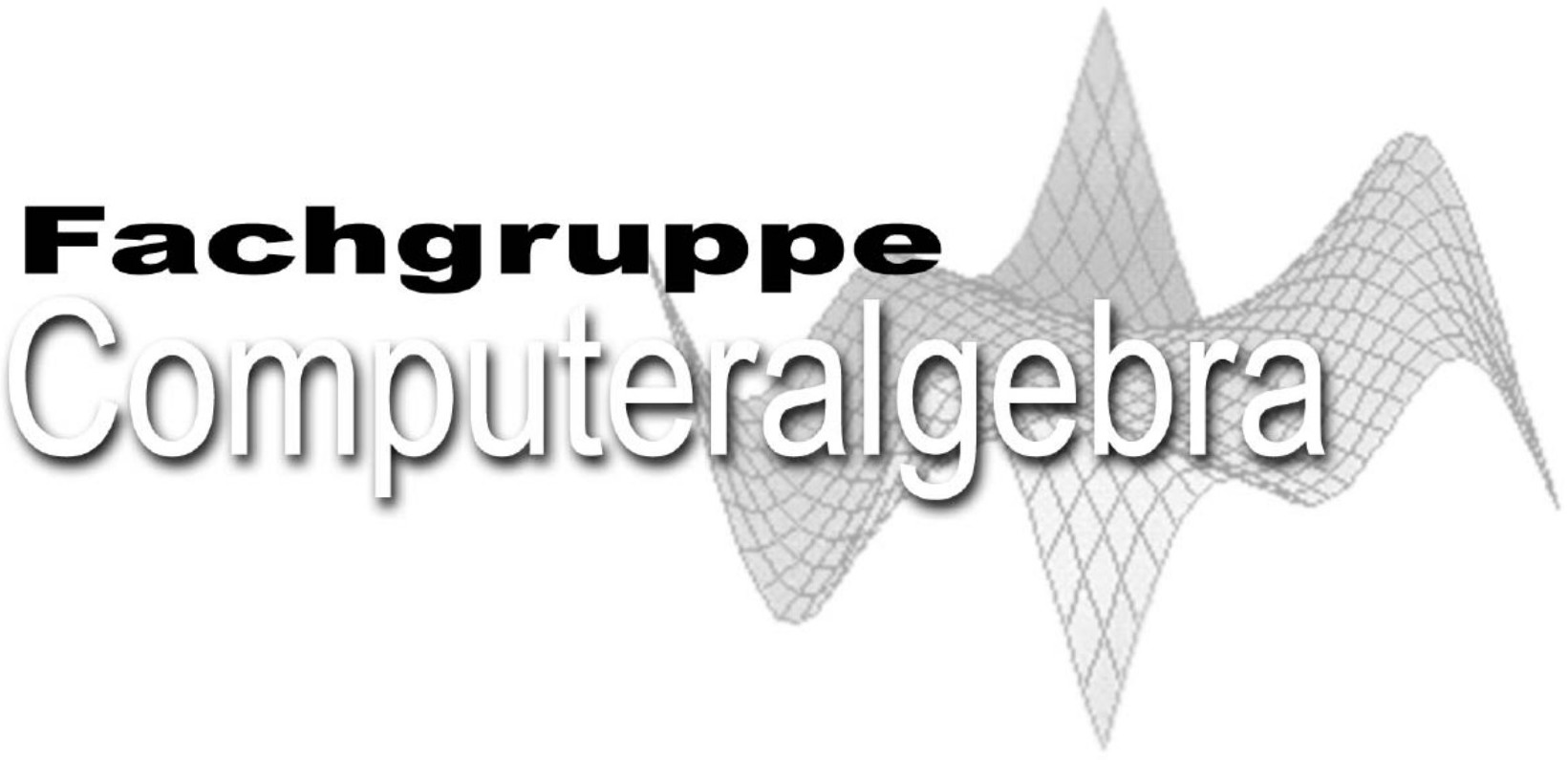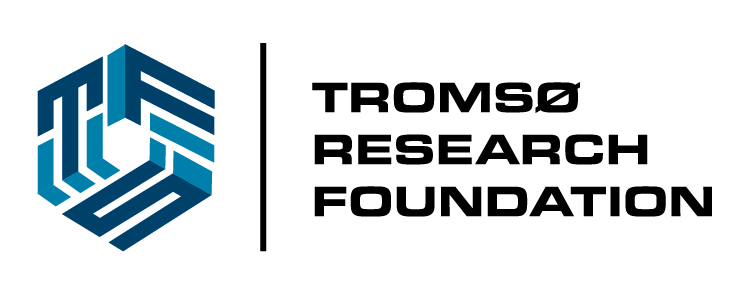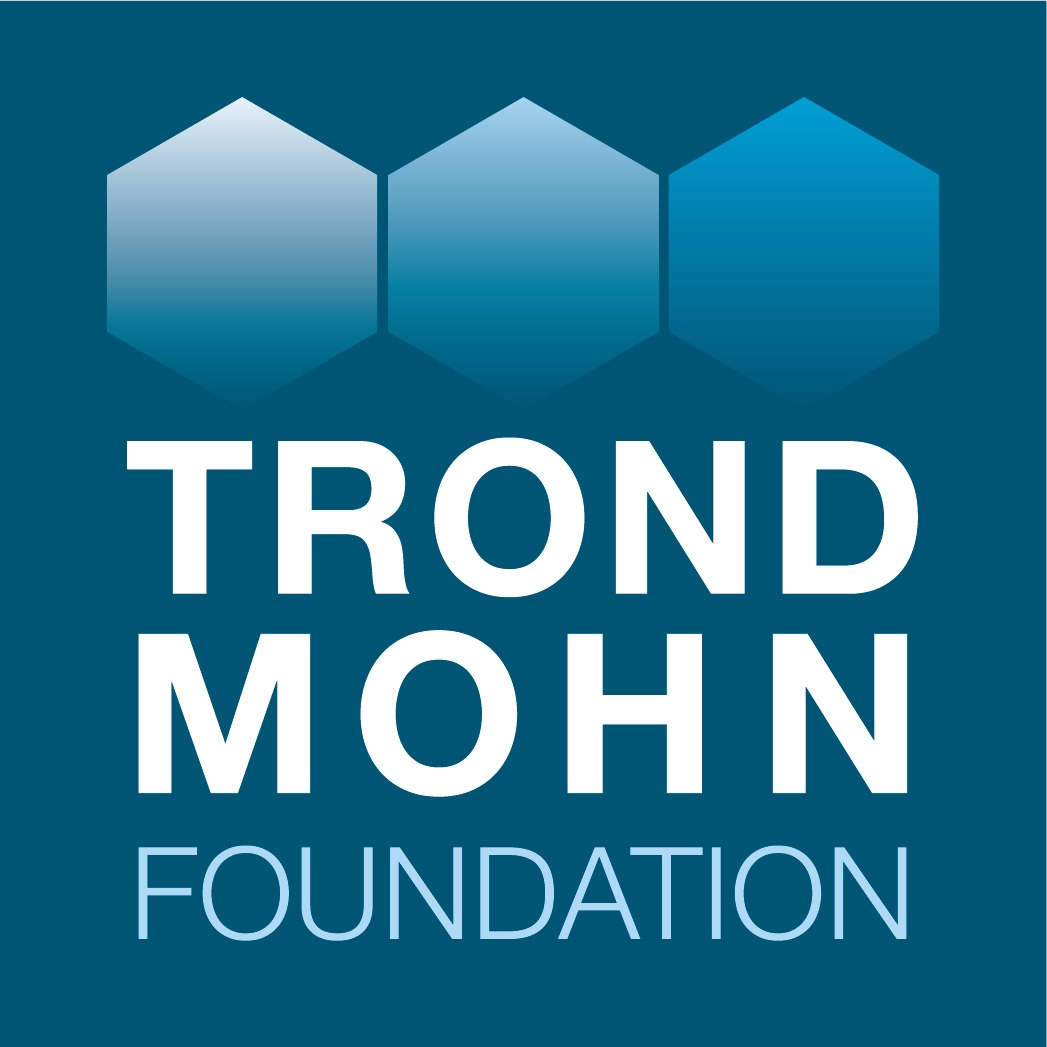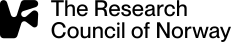# Invited Speakers

## Titles and Abstracts

Nikhil Srivastava
UC Berkeley, USA### The Complexity of Diagonalization

Abstract: Despite its fundamental nature, the question of designing a provably efficient and correct algorithm for approximately diagonalizing a complex matrix remains unresolved. We survey various approaches to this question over the past five decades using tools from complex analysis, differential geometry, numerical analysis, random matrix theory, and symbolic computation and report on some recent progress. A key ingredient in the proofs is the fact that a tiny random perturbation of any matrix behaves more or less like a normal matrix in a certain quantitative sense.

Rekha R. Thomas
University of Washington, USA### Two Views of P3

Abstract: Image formation in a pinhole camera is foundational to computer vision. Based in classical projective geometry and inspired by paintings and photogrammetry, the first problems in this area date back to the 1800s. I will describe some of the main algebro-geometric questions and answers that arise in the context of two cameras. Classical algebraic geometry and invariant theory come up naturally in this setting.

Frank Vallentin
Universität zu Köln, Germany### Least Distortion Euclidean Embeddings of Flat Tori

Abstract: Lattices (discrete subgroups of \$n\$-dimensional Euclidean spaces) are ubiquitous objects in mathematics.

One typical algorithmic problem for lattices is the closest vector problem (given a lattice and a point in Euclidean space, which lattice vector is closest to this given point?). In general the closest vector problem is NP-hard. One potential approach to derive efficient approximation algorithm the closest vector problem could go via the concept of least distortion metric embeddings.

In this talk I will derive and analyze an infinite-dimensional semidefinite program which computes least distortion embeddings of flat tori \$R^n/L\$, where \$L\$ is an \$n\$-dimensional lattice, into Hilbert spaces. This enables us to provide a very simple, constant factor improvement over the previously best known lower bound on the minimal distortion of an embedding of an \$n\$-dimensional flat torus. As further applications we prove that every \$n\$-dimensional flat torus has a finite dimensional least distortion embedding, and we derive some optimal embeddings of flat tori given by certain important lattices.